# pseudodifference

## Pseudodifference of filter objects ★★

Author(s): Porton

Let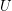is a set. A filter(on) is a non-empty set of subsets ofsuch that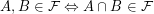. Note that unlike some other authors I do not require.

I will call the set of filter objects the set of filters ordered reverse to set theoretic inclusion of filters, with principal filters equated to the corresponding sets. See here for the formal definition of filter objects. I will denote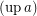the filter corresponding to a filter object. I will denote the set of filter objects (on) as.

I will denote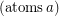the set of atomic lattice elements under a given lattice element. Ifis a filter object, thenis essentially the set of ultrafilters over.

Problem   Which of the following expressions are pairwise equal for allfor each set? (If some are not equal, provide counter-examples.)
\item;

\item;

\item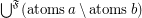;

\item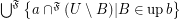.

Keywords: filters; pseudodifference